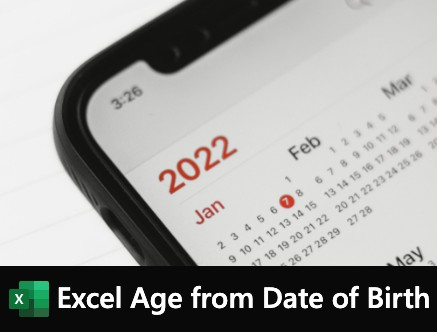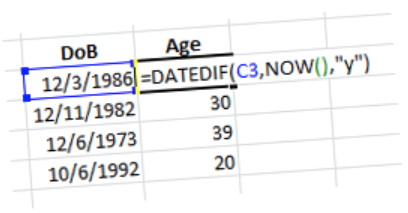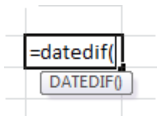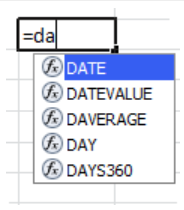### Excel Age from Date of Birth## Excel Age from Date Of Birth

Excel Calculating Age from Date of Birth – It’s easy to calculate someone’s age from their date of birth if you know about Excel’s DATEDIF function, unfortunately it’s easy to miss this function as it is not documented. I am baffled as to why it is never included in the list of Excel functions.

To have a formula that updates itself as time goes on you need to get the current date into your formula and you can do this using either the NOW or TODAY functions. There’s no substantial difference between the two functions for this calculation; NOW gives you the current date and time, whereas TODAY just gives the current date. When you enter the function into your formula remember to include the brackets like this, NOW() or TODAY().So, to calculate someone’s age in years make sure that you have entered their date of birth correctly as a proper Excel date (using slashes or dashes) and then enter the formula:

=DATEDIF(C3,NOW(),”y”)

Where C3 is the cell containing the date of birth. The interval value “y” must be entered in double quotation marks. The age is calculated in whole calendar years and will update on the subject’s birthday. If you want to calculate the exact age and include the months or even the days then you have to change the interval value. Refer to the table of interval values below for further details.

## Sample DATEDIF Formulas

To calculate the age in months for the current year, the formula is:

=DATEDIF(C3,NOW(),”ym”)

And therefore, to state the age in this type of format: “24 years, 8 months” the formula is:

=DATEDIF(C3,NOW(),”y”) &” years, “&DATEDIF(C3,NOW(),”ym”)&” months.”

No! Don’t type it all in, copy and paste it from here. I always do, life’s too short to have to reinvent formulas like this.

## The DATEDIF Worksheet Function

The function is not documented so I am going to have to be a bit boring and do the documentation here in case you want to Bookmark this page. I always find the interval values a bit difficult to remember; “y” for year is dead easy but I struggle to remember the others. The only time you see any indication that DATEDIF exists is when you type it into a cell and Excel pops up a ghostly apparition as you enter the first bracket.This function is available in all versions of Excel since way back:

=DATEDIF(Date1, Date2, Interval)

Date1 is the first date, Date2 is the second date. Date1 should be before Date2 in the calendar. Interval is the interval type and must be one of the values from the following table:

## Interval Values for the DATEDIF function

Interval Description
m
Complete Calendar Months
d
Number of Days
y
Complete Calendar Years
ym
Months excluding Years
yd
Days excluding Years
md
Days excluding Years and Months

The first three interval values give the gross number of years, months or days between the two dates and the last three interval values work out the days and months as if the dates were in the same year and are very useful for calculating the fragments of months and days where the date difference needs to be calculated precisely.

You must enclose the interval in double quotation marks if you are including it directly in the formula or you can enter it into a cell without quotation marks and use the cell reference of the cell in the formula instead.

If Date1 is later than Date2, DATEDIF returns a #NUM! error. If either Date1 or Date2 is not a valid date, DATEDIF returns a #VALUE! error. If Interval is not one of the items listed in the table, DATEDIF returns a #NUM! error.

## Watch the VideoTo get the best out of Excel formulas you’ll need to have a good, working knowledge of Excel functions. Come along on our dedicated Excel functions course and discover what Excel formulas can do for you.

Call 020 7920 9500 now for details.